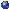## Events

##### Junior GeometryJoakim Asger F?rgeman, Zoom: Gaitsgory\'s construction of central sheaves on the affine flag variety
Tuesday, March 09, 2021, 03:45pm - 04:45pm
Let G be a (split) connected reductive group over a finite field \$F_q\$, and let \$K=F_q((t))\$, \$O=F_q{t}\$. Then a theorem of Bernstein realizes the bi-\$G(O)\$-invariant compactly supported functions on \$G(K)\$ as the center of the algebra of bi-\$I\$-invariant compactly supported functions on \$G(K)\$, where \$I\$ denotes the Iwahori subgroup of \$G(O)\$.In this talk we are going to talk about Gaitsgory's geometric version of this result. More precisely, using nearby cycles, Gaitsgory constructed a tensor functor from the symmetric monoidal category of \$G(O)\$-invariant perverse sheaves on the affine grassmannian to the center of the monoidal category of Iwahori-invariant perverse sheaves on the affine flag variety. Moreover, this functor satisfies a myriad of nice properties, some of which are invisible at the level of Hecke algebras.Location: Zoom